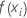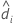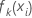# Fits and probabilities for Fit Model and Discover Key Predictors with TreeNet® Classification

###### Note

The expression of the relationship between the fits and the probabilities depends on the number of levels in the response variable:

## Binary response

The fitted value for the ith row with the optimal model is in terms of the odds:

where the odds have their customary definition:

The following relationship converts the fitted values into the probability for the event:
The probability for the non-event has the customary form:

## Multinomial response

The fitted value for the ith row with the optimal model has the following form:

The following relationship converts the fitted values into the probability for one of the outcomes, k.

For example, the probability for the outcome coded as 1 from a multinomial response with three levels has the following form:

## Notation

TermDescriptionfitted value for the ith rowfitted odds for the eventfitted value for the ith row for the kth level of the response variable